# OFDM Channel Estimation (2) - Comb-Type pilots¶

In the previous notebook, we have used the Schmidl&Cox metric to estimate and compensate a carrier frequency offset. However, given that there is noise, the S&C CFO estimation can never be perfect and a residual CFO remains.

As a rough approximation, a small frequency offset essentially creates a time-variant channel, where the phase of the channel changes over time. In this notebook, we will look at methods to compensate this time-variant behaviour of the channel, which is not achievable with the block-type estimation.

Let us quickly go through some mathematical fundamentals. From the previous notebook we know that a residual CFO $\Delta_f$ can be modeled as

$$y(t)=x(t)\exp\left(j2\pi \Delta_f t\right),$$

i.e. the received signal is multiplied by complex exponential. Now, let's assume that $\Delta_f T$ is very small, where $T$ is the length of one OFDM symbol. Hence, we assume that over the duration of one OFDM symbol, the channel is assumed to be static. However, when multiple OFDM symbols occur after each other, each OFDM symbol experiences a different starting phase, as illustrated below:

In :
# Source code has been redacted in online version
# Omitting 15 lines of source code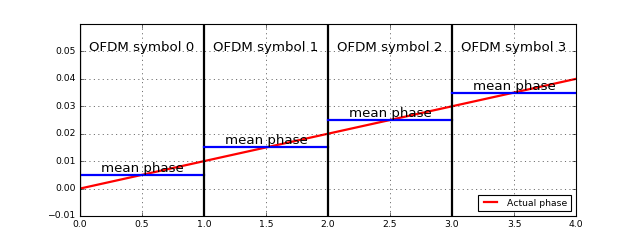As we see, adjacent OFDM symbols experience a different average phase rotation due to a small CFO. In the following we will look at methods how to compensate this phase shift.

In :
# Source code has been redacted in online version
# Omitting 7 lines of source code


Also, we import OFDM functional blocks including the CFO extraction and compesation blocks that we defined in the previous notebook:

In :
# Source code has been redacted in online version
# Omitting 5 lines of source code


## Recap of the block-type channel estimation¶

Let us quickly recap the block-type channel estimation: In the block-type pilot scheme, the channel was estimated at the beginning of the block and then used for equalization in every following OFDM symbol. Hence, it could not represent any time-variance. Below, we will look a bit more closly at what happens with a residual CFO and block-type pilots.

Let's write a generic function for the OFDM transmission that we will use throughout this notebook.

In :
def runTransmissionWithCFOCorrection(ofdm, transmitObjFunc, channelFunc, rxObjFunc, B=441*5*4, Fc=8000, cfo=0, bypassCFO=False):
frameLen = getattr(ofdm, 'frameLen', (ofdm.K+ofdm.CP) * (1+1+ofdm.Npayload))

# Create the components
env = Environment(samplerate=44100)

# transmitter
tx = transmitObjFunc(env, ofdm)

# channel
chan = PassbandChannel(env, channelFunc=channelFunc, Fc=Fc, B=B, cfo=cfo)

# synchronization
scMetric = SchmidlCoxMetric(env, ofdm=ofdm, minEnergy=0.1)
scDetect = SchmidlCoxDetectPeaks(env, K=ofdm.K, CP=ofdm.CP, frameLen=frameLen)
cfoExtract = CFOExtractor(env, ofdm, rate=B)
cfoCorrect = CFOCorrection(env, rate=B, bypass=bypassCFO)  # allow to turn off the CFO correction for testing purposes

rx = rxObjFunc(env, ofdm)

# visualization
fig = env.figure(figsize=(10,6))
gs = GridSpec(3,2)
showSpectrum = PlotSpectrum(env, windowDuration=0.3, logScale=1, axes=fig.add_subplot(gs[0,:]), xlim=(0, 44100/2), ylim=(-100, -40))
showCFO = PlotWaveform(env, axes=fig.add_subplot(gs[1,0]), integerXaxis=True, windowDuration=100, ylim=(-5,5), title='Measured CFO')
showHest = PlotBlock(env, axes=fig.add_subplot(gs[2,0]), numLines=2, ylim=(-4,4), title='Channel Estimation')
showConstellation = PlotConstellation(env, axes=fig.add_subplot(gs[1:,1]), title='Rx Constellation', numLines=5, xlim=(-1.5,1.5))

# set up the connections
tx.transmitsTo(chan)
chan.transmitsTo(showSpectrum, stream='RF')
chan.transmitsTo(scMetric)

scMetric.transmitsTo(scDetect)

scDetect.transmitsTo(cfoExtract, stream='P_metric')
scDetect.transmitsTo(cfoCorrect, stream='frame')

cfoExtract.transmitsTo(showCFO, stream='CFO')
cfoExtract.transmitsTo(cfoCorrect, stream='CFO')

cfoCorrect.transmitsTo(rx, stream='frame')

rx.transmitsTo(showHest, stream='Hest')
rx.transmitsTo(showConstellation, stream='constellation')

env.run()


The function expects functions to create the transmitter, channel and OFDM demodulator objects and then creates a visualization of the signals and connects all blocks.

For subsequent simulations, we define a common OFDM parameter structure:

In :
ofdm = OFDM(K=256, Kon=201, CP=64, Npayload=5, qam=16)
ofdm.frameLen = (256+64)*(7)


Let's run it with block-type channel estimation with a residual CFO. We simulat the residual CFO by applying a small CFO and bypassing the CFO correction:

In :
runTransmissionWithCFOCorrection(ofdm,
BlockPilotRandomDataOFDMTransmitter,
lambda env: SimulatedChannel(env, MultipathChannelEffect(taps=[(0,1), (0.0005, 0.9)])),
lambda env, ofdm: BlockPilotOFDMReceiver(env, ofdm, BlockPilotLSChannelEstimator(ofdm)),
B=441*5*4, Fc=10000, cfo=0.4, bypassCFO=True)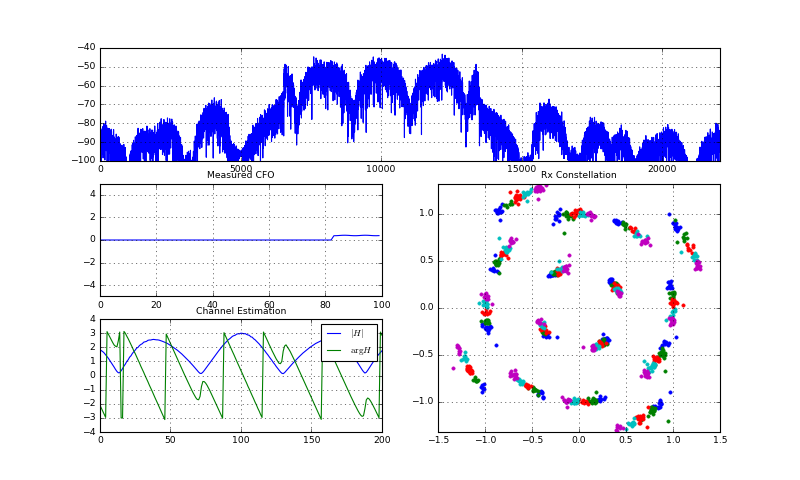Stop received from external


As we see, the constellation appears rotated. Examining more closely, we see that the points with the same colors (indicating the different OFDM symbols) gather in clusters. This confirms that each OFDM symbol experiences a different average phase, and hence the constellation is rotated according to this average phase. Now, let's look at what happens when we use the audio channel:

In :
# Source code has been redacted in online version
# Omitting 5 lines of source code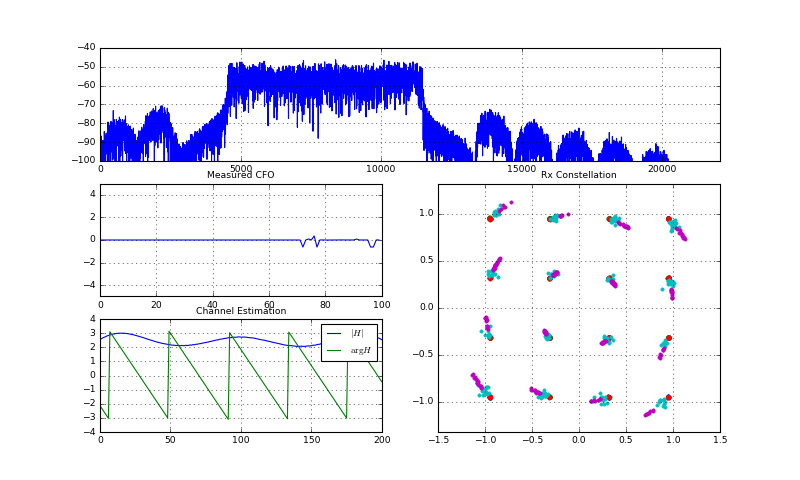Stop received from external


Depending on your actual soundcard quality and speakers (above was obtained with a cable between speaker output and microphone input), you will see sometimes phase rotations of the constellation diagram that look quite similar to our simulation before. Again, we see that it should be beneficial to apply a dedicated equalization to each OFDM symbol seperately.

Below, we will examine two techniques for dedicated treatment of each block. First, we start with a single pilot for phase correction, but keep the block-type pilot insertion. Second, we will switch over to real comb-type pilot insertions.

## Common Phase Error Compensation¶

We have seen before, that each OFDM symbol experiences a different phase shift. Hence, if we would rotate the constellation symbols for each OFDM symbol by this phase shift, we will end up with correct constellation diagrams. To know the phase shift, we simply insert a single pilot carrier into each OFDM symbol and examine its phase rotation. For simplicity, we take the value $1+0j$ as the pilot value. Let's write an according transmit block:

In :
# Source code has been redacted in online version
# Omitting 6 lines of source code


Let's look at the transmitted constellation diagram:

In :
# Source code has been redacted in online version
# Omitting 11 lines of source code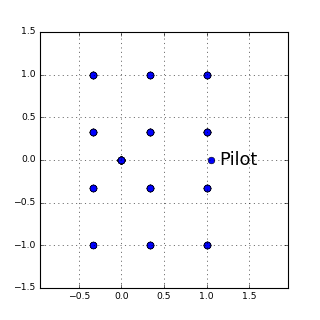OK, there's the pilot appearing at the value $1+0j$ marked in the constellation diagram. Now, let's artificially apply a small CFO to the signal and look where the pilots occurs:

In :
# Source code has been redacted in online version
# Omitting 15 lines of source code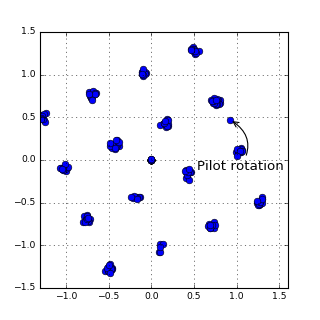Pilot rotated to 0.93+0.47j


Ah, the pilot occurs phase rotated from $1+0j$ to $0.95+0.39j$. With this information, we can now multiply the constellation diagram by the corresponding inverse phase to obtain a nice constellation diagram:

In :
# Source code has been redacted in online version
# Omitting 7 lines of source code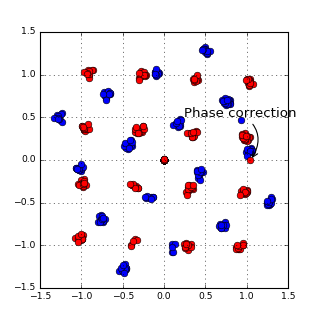Clearly, the equalized (red) constellation has the correct rotation now. Now, that we have checked the technique offline, let's integrate the single pilot into the transmission chain. As we have already defined the transmitter above, we can directly move on to the demodulator:

In :
class BlockPilot1PilotOFDMReceiver(BlockPilotOFDMReceiver):
scPreamble, pilotBlock, symbols = self._extractParts(frame)
self._send(pilotBlock, stream='preamble')  # forward debug information

# call the channel estimation
Hest = self._channelEstimator.estimate(pilotBlock)
self._send((3*abs(Hest)/max(abs(Hest)), np.angle(Hest), dict(legend=["$|H|$", "$\\arg H$"])), stream='Hest')

constellations = []
for S in symbols:
un_eq = np.fft.fftshift(np.fft.fft(S))[self._carrierIndices]
equalized = un_eq / Hest

# Compensate common phase error based on the single pilot
pilotPos = len(equalized) // 2    # Extract the pilot from the carriers
pilot = equalized[pilotPos]
equalized /= (pilot/abs(pilot))   # Compensate the phase rotation of the pilot

constellations.append(equalized)

self._send(constellations, stream='constellation')


Let's use this receiver in a simulation where we have a residual CFO:

In :
# Source code has been redacted in online version
# Omitting 5 lines of source code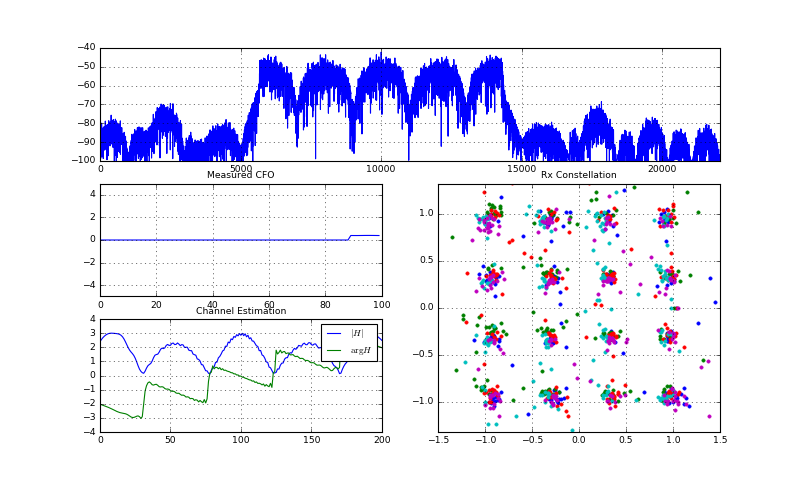Stop received from external


Great! Even though we have a small CFO, we still obtain correctly positioned constellation points due to the single pilot used for the common phase correction.

Now, let's move on and check out the behaviour in the real audio channel:

In :
# Source code has been redacted in online version
# Omitting 5 lines of source code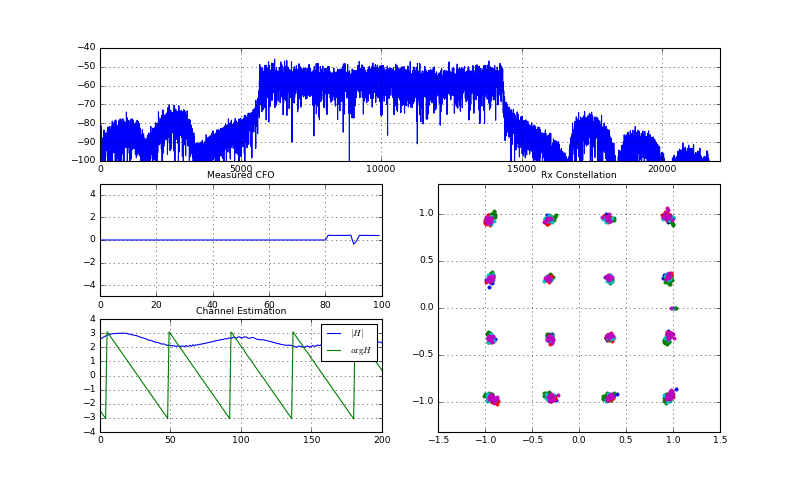Stop received from external


Also in the audio channel, we see the pilot is doing its job (note that we have added an artificial CFO). Moreover, in case it happens that the constellation gets messed up due to the soundcard impairments, still the constellation points occur as arcs centered at the correct constellation points. Now, that we have already integrated one pilot into each OFDM symbol, the question arises, why we wouldn't put the full pilot information into each symbol, such that we can actually calculate a channel estimate for each OFDM symbol. This comb-type pilot scheme is discussed in the next section.

## Comb-Type Pilot Insertion¶

With comp-type pilots, each OFDM symbol contains both data and pilots, where the pilots occur on every $x$th carrier. With this method, the pilots experience exactly the same channel conditions as the data, and hence the channel estimate is most up-to-date. This is in large contrast to block-type pilots, where the channel is estimated once and then used for every subsequent OFDM symbol.

Let's go ahead and first implement the OFDM transmitter with comb pilots. First, we create a utility function that calculates the data and pilot carrier index given the OFDM parameter structure:

In :
def calcPilotAndDataCarriers(ofdm):
assert (ofdm.Kon % ofdm.pilotSpacing == 1)  # make sure we can have a pilot at both edges of the Kon allocated carriers.

# the pilot carriers occur every pilotSpacing carriers
pilotCarriers = np.arange(ofdm.Kon // ofdm.pilotSpacing + 1)*ofdm.pilotSpacing

# the data carriers are all other carriers
dataCarriers = np.delete(np.arange(ofdm.Kon), pilotCarriers)
return pilotCarriers, dataCarriers


Let's try this function with some dummy data:

In :
# Source code has been redacted in online version
# Omitting 7 lines of source code

Pilots: [0 4 8]
Data  : [1 2 3 5 6 7]OK, the function works and returns the expected pilot and data carrier allocation. For the above example, we have 9 carriers and a pilot spacing of 4, which inserts a pilot every 4 carriers and leaves 3 data carriers between the pilots.

We can now go ahead and implement the comb-type transmitter. As the pilot values we again use the Zadoff-Chu sequence as we used for the block-type pilots.

In :
# Source code has been redacted in online version
# Omitting 25 lines of source code


At the receiver side, we implement a simple receiver that applied ZF equalization. However, the actual channel estimation is delegated to a dedicated object which we will develop below. For now, let's just assume it's already there:

In :
class CombPilotOFDMReceiver(Component):
def __init__(self, env, ofdm, channelEstimator):
super().__init__(env, None)

self._ofdm = ofdm
self._channelEstimator = channelEstimator    # store the channel estimation object for later use

self._usefulCarriers = get_carrierIndices(ofdm)
self._pilotCarriers, self._dataCarriers = calcPilotAndDataCarriers(ofdm)

scPreamble, symbols = self._extractParts(frame)
self._send(scPreamble, stream='preamble')  # forward debug information

# Treat each OFDM symbol separately
eqs = []
for S in symbols:
un_eq = np.fft.fftshift(np.fft.fft(S))[self._usefulCarriers]

# call the channel estimation object
Hest = self._channelEstimator.estimate(un_eq)

# use ZF equalization
eq = un_eq / Hest
eqs.append(eq[self._dataCarriers])
self._send((abs(Hest)/max(abs(Hest))*3, np.angle(Hest)), stream='Hest')
self._send(eqs, stream='constellation')

def _extractParts(self, frame):
"""This function extracts the preamble, pilotBlock and payload symbols from the received frame, given the parameters
of the ofdm structure."""
CP, K = self._ofdm.CP, self._ofdm.K

scPreambleStart = CP
scPreamble = frame[scPreambleStart:scPreambleStart+K]

symbols = []
symStart = (CP+K)+ CP + s*(CP+K)
symbols.append(frame[symStart:symStart+K])

return scPreamble, symbols


Now, given that we have already implemented the receiver, we can focus on the actual channel estimation algorithm. To understand the comb-type estimation technique, we'll do some offline-experiments. First, we generate an artificial receive signal and plot the channel frequency response and the received spectrum:

In :
# Source code has been redacted in online version
# Omitting 18 lines of source code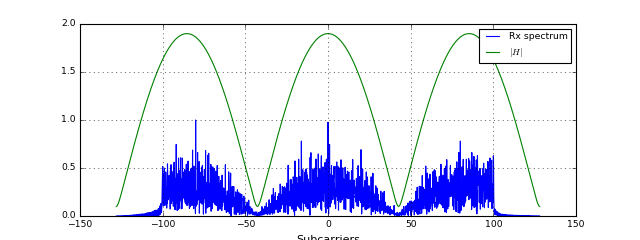Clearly, we experience a frequency-selective channel. Let's now look at the channel estimation at the pilot carriers:

In :
# Source code has been redacted in online version
# Omitting 17 lines of source code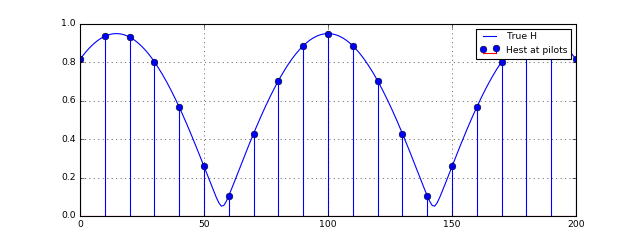OK, the channel is correctly estimated at the pilots. However, we are actually interested in the channel at the data carriers. Hence, we have to use some interpolation technique to interpolate between the measurements at the pilot carriers. Below, we write a function to illustrate the interpolation in different domains.

In :
from scipy.interpolate import interp1d

def showChannelInterpolation(ofdm, ofdm_signal, h, t_offset=0, interpolation='linear'):
# t_offset denotes the error of the coarse time-synchronization

# extract time-domain symbol and carrier indices
symbol0 = ofdm_signal[ofdm.CP+ofdm.K+ ofdm.CP +np.arange(ofdm.K) - t_offset]
usefulCarriers = get_carrierIndices(ofdm)
pilotCarriers, dataCarriers = calcPilotAndDataCarriers(ofdm)
S_uneq = np.fft.fftshift(np.fft.fft(symbol0))[usefulCarriers] / np.sqrt(ofdm.K)
rx_pilots = S_uneq[pilotCarriers]

# obtain the true channel response
H_true = np.fft.fftshift(np.fft.fft(np.hstack([np.zeros(t_offset), h]), ofdm.K))[usefulCarriers] / 2

# channel estimation and interpolation
Hest_at_pilots = rx_pilots / tx._pilotVals   # estimation at pilot carriers
Hest = Hest_fun(np.arange(ofdm.Kon))         # evaluate interpolation at data carriers

# plotting of results
plt.figure(figsize=(10,5))
plt.subplot(221); plt.title('Absolute value')
plt.stem(pilotCarriers, abs(Hest_at_pilots), label='H estimated at pilots')
plt.plot(abs(H_true), label='H')
plt.plot(abs(Hest), label='H estimated and interpolated', lw=2)
plt.grid(True); plt.legend(loc='best');

plt.subplot(223); plt.title('Phase')
plt.stem(pilotCarriers, np.angle(Hest_at_pilots), label='H estimated at pilots')
plt.plot(np.angle(H_true), label='H')
plt.plot(np.angle(Hest), label='H estimated and interpolated', lw=2)
plt.grid(True); plt.legend(loc='best');

plt.subplot(122); plt.title('In complex plane')
plt.plot(Hest_at_pilots.real, Hest_at_pilots.imag, 'ro', label='H estimated at pilots')
plt.plot(H_true.real, H_true.imag, label='H')
plt.plot(Hest.real, Hest.imag, label='H estimated and interpolated')
plt.grid(True); plt.legend();


Let's show the interpolation effect using a linear interpolation with our channel. For now, let's assume the synchronization was exact (i.e. t_offset=0):

In :
showChannelInterpolation(ofdm, ofdm_signal_rx, h=h, interpolation='linear', t_offset=0)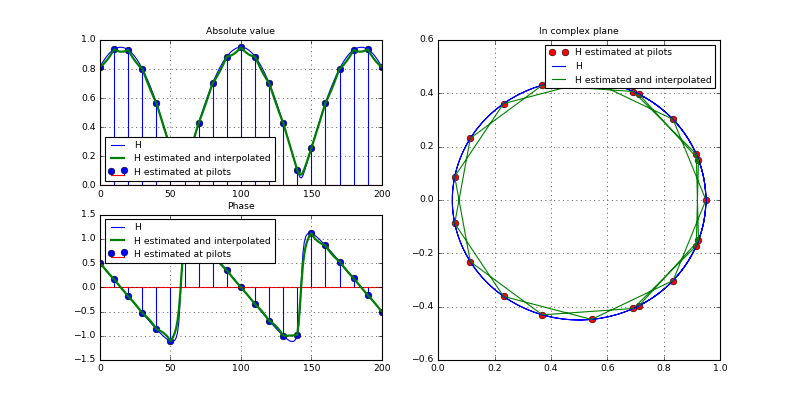How can we interpret above plots? First, let's look at the magnitude plot: We see a strange behaviour of the interpolation: Somehow, it occurs that the channel is estimated too small? For the phase estimation it looks better.

The reason for this behaviour can be seen in the right-most plot. There, the locus of the actual channel is plotted in the complex plane. Moreover, the red dots indicate the pilot measurements and the green line shows the interpolation values. There, we clearly see that the linear interpolation between the pilots does not really reflect the actual channel values. Let's try a different, more complex, interpolation:

In :
showChannelInterpolation(ofdm, ofdm_signal_rx, h=h, interpolation='quadratic')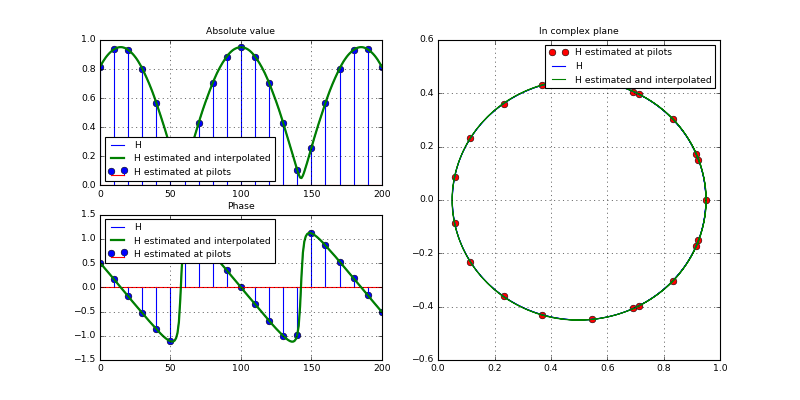Great! With the quadratic interpolation (i.e. 2nd order spline), the estimated and interpolated channel is perfectly on top of the real channel. So, let's use quadratic interpolation and go ahead and implement the first version for the chanel estimator:

In :
from scipy.interpolate import interp1d

class CombPilotChannelEstimator(object):
def __init__(self, ofdm):
self._ofdm = ofdm

# pre-calculate pilot values and positions
self._pilotCarriers, _ = calcPilotAndDataCarriers(ofdm)

def estimate(self, un_eq):
# extract the pilots
rxPilots = un_eq[self._pilotCarriers]

# estimation and interpolation
Hest = rxPilots / self._pilotVals  # estimation at pilot carriers

# interpolation between the carriers
k = np.arange(self._ofdm.Kon)
return Hest_interp(k)


That's pretty straigt-forward, isn't it? Let's try out our chain with the comb-type pilots. First, we work with the simulated channel:

In :
ofdm2 = OFDM(K=256, Kon=201, CP=64, Npayload=5, pilotSpacing=10, qam=16)